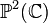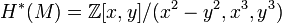# Connected sum of two complex projective planes with same orientation

(diff) ← Older revision | Latest revision (diff) | Newer revision → (diff)
Jump to: navigation, search
This article is about a particular topological space (uniquely determined up to homeomorphism)|View a complete list of particular topological spaces

## Definition

This topological space is defined as the connected sum of two copies of the complex projective plane$\mathbb{P}^2(\mathbb{C})$, where they are glued with the same orientation.

## =Homology groups

The homology groups are as follows:

$Z}, & \qquad p = 0,4 \\ \mathbb{Z} \oplus \mathbb{Z}, & \qquad p = 2 \\ 0, & \qquad \operatorname{otherwise}\\\end{array$

### Cohomology groups

The cohomology groups are as follows:

$Z}, & \qquad p = 0,4 \\ \mathbb{Z} \oplus \mathbb{Z}, & \qquad p = 2 \\ 0, & \qquad \operatorname{otherwise}\\\end{array$

The cohomology ring is as follows:$H^*(M) = \mathbb{Z}[x,y]/(x^2 - y^2, x^3,y^3)$

### Homotopy groups

The fundamental group is the trivial group.